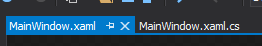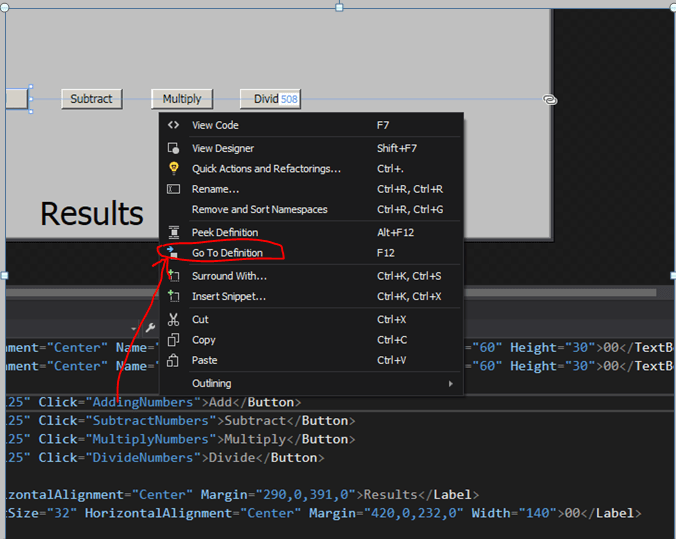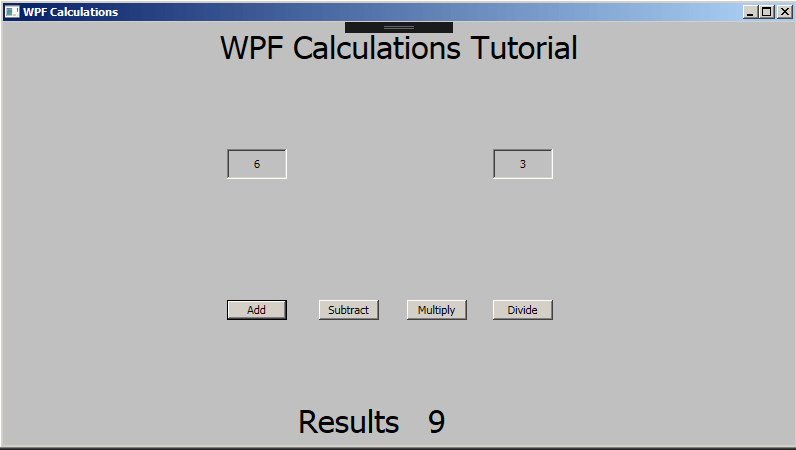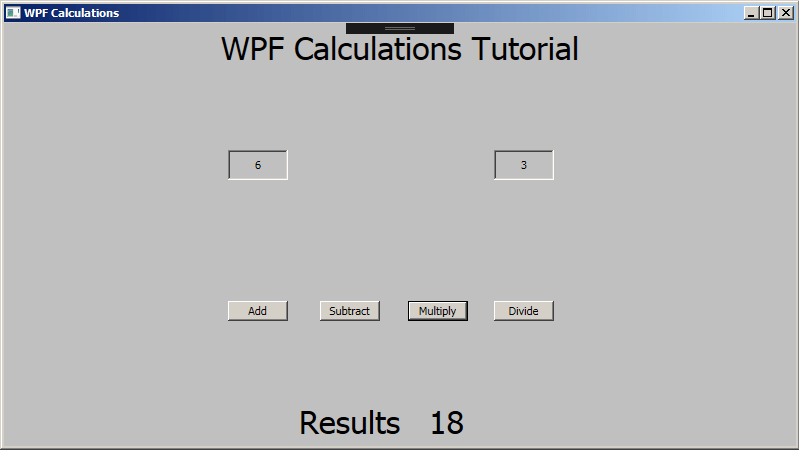# WPF C# Tutorial – Making a Calculator App in Visual Studio

With all the buttons been added to the screen now its time to add some events to the buttons. We can directly add the events to the buttons from the XML file.

Time to add some events to the form.

Notice that we have added a new property called Click = “AddingNumbers” inside the button tag. This means when the button will be clicked it will trigger that adding number function.

The other buttons have similar events attached to them. Make sure you have noted them down as you can see here because they are case sensitive.To check that our C# script is available to us, see the tab on top right under the tool bar click on the MainWindow.xaml.cs tab and it should open up the c# script that is attached to this app right now.

Above is the empty C# script. All the codes in this script are the default ones and we haven’t added anything new to it yet. So lets start adding all of the events to this. To begin with that go back to the mainWindow.XAML file from the tag and do the following –Earlier we added Click events to the buttons, right click on the AddingNumbers text and click on Go to Definition. Once you done that it will add the events to the C# script. Do the same for other events and it will add them.

For some reason it if does not add the events automatically you can type them in there.

In the code above all of the events has been added to the code. Now we will add some crucial code to make this calculator work.

First we will need to add 3 public variables. All these variables will be the double data types. The reason we are using double data types is because we can use decimal points. In integers we can only use whole numbers for example 1, 2, 3 or 100 but when we divide numbers they will often return numbers that are 3.4, 7.12 or so on. Integers will not accept decimal numbers therefore we will be using decimal numbers.

Above is the adding numbers function. This function will trigger when the add button is clicked. Note – add the green texts are the comments. They are used enhance our understanding of the code.

num1 = Convert.ToDouble(numberA.Text); This line is converting the text from the number A text box into a double data type and storing it inside the num1 variable.

num2 = Convert.ToDouble(numberB.Text); This line is converting the text from the number B text box into a double data type and storing it inside the num2 variable.

We will then use the num1 and num2 to do our calculations. After we have captured the input from both of the text boxes we can use the total number variable and add both num1 and num2 together.

totalNumber = num1 + num2; This line of code will add both num1 and num2 together and store them inside the total number variable.

Finally we need to show the results on the screen. We already have a label called results now we will need to link the total number variable to the results label.

result.Content = totalNumber.ToString(); This line will find the label called result and add the total number content to it. The reason we are using the ToString() end of the total number variable. The to string command means that it will take the values from the total number variable to convert it to string meaning text so we can show it on the form display.We are adding 6 and 3 together. The results show 9. The adding function is working.

Above is the subtract numbers function. This function will trigger when the Subtract button is clicked. You will notice it is very similar to the function we did before. The only different is this line below –

totalNumber = num1 – num2; // substract the two numbers together and place them in the total number variable

The total number is now subtracting num1 and num2. We are using the minus sign instead of the plus sign in this code. The rest of the code is the same.We are subtracting 6-3 and the result is showing 3.

Above is the multiply numbers function. Once again it is the same as the ones before except for the arithmetic symbols.

totalNumber = num1 * num2; // multiply the two numbers together and place them in the total number variable

The total number is now multiplying the two numbers together notice that we are using a asterisk symbol to complete the multiplication for this task.

Rest of the code can stay the same.We are multiplying 6 X 3 and result is 18.

Above is the divide numbers function. For this function we kept most of the code similar to the last 3 functions except for two difference. The first different is the symbol for divide we are using slash symbol for the division.

totalNumber = num1 / num2; // divide the two numbers together and place them in the total number variable

Since division usually can generate decimal point numbers our answers might return something line 7.3333333 or bigger we need round down the numbers to maximum two decimal points.

totalNumber = Math.Round(totalNumber, 2); // round the numbers down 2 decimal points

The line above is using the Math.Round function which will take the total number variable and round it down to 2 decimal points and store it back into the total number variable.Now finally we are dividing 8 / 3, the result is showing 2.67 there would have been more decimal points but we are rounding the decimal points down to 2 maximum. So all that works. Well done.

That will be all for this calculator app. Well done you’ve followed this tutorial so far.